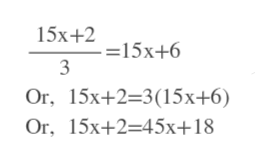Solve the equation and check your solution. (Some equations have no solution. If there is no solution, enter NO SOLUTION.)15x + 2  .   = 1 5x + 6           3

Question

Solve the equation and check your solution. (Some equations have no solution. If there is no solution, enter NO SOLUTION.)

 15x + 2  .   = 1 5x + 6           3

Step 1

First cross multiply by 3 and dist...help_outlineImage Transcriptionclose15х+2 15х+6 3 Or, 15х+2-3(15х+6) Or, 15x+2-45х+18 fullscreen

Want to see the full answer?

See Solution

Want to see this answer and more?

Our solutions are written by experts, many with advanced degrees, and available 24/7

See Solution
Tagged in

Equations and In-equations